# Formula Auditing

Formula auditing in Excel allows you to display the relationship between formulas and cells. The example below helps you master Formula Auditing quickly and easily.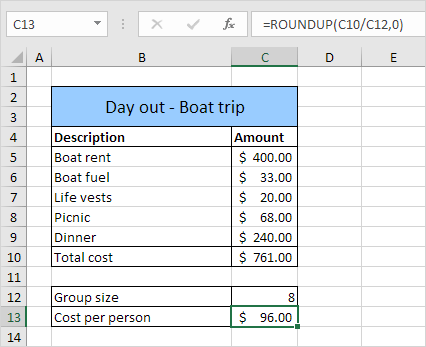### Trace Precedents

You have to pay \$96.00. To show arrows that indicate which cells are used to calculate this value, execute the following steps.

1. Select cell C13.

2. On the Formulas tab, in the Formula Auditing group, click Trace Precedents.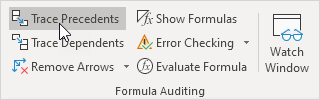Result: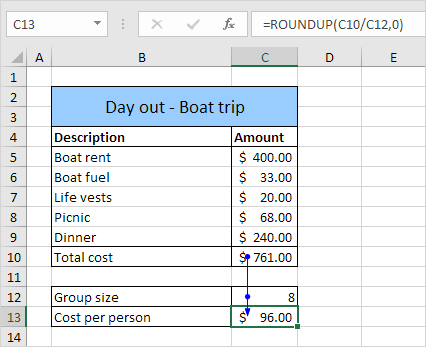As expected, Total cost and Group size are used to calculate the Cost per person.

3. Click Trace Precedents again.As expected, the different costs are used to calculate the Total cost.

### Remove Arrows

To remove the arrows, execute the following steps.

1. On the Formulas tab, in the Formula Auditing group, click Remove Arrows.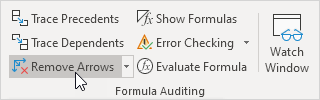### Trace Dependents

To show arrows that indicate which cells depend on a selected cell, execute the following steps.

1. Select cell C12.

2. On the Formulas tab, in the Formula Auditing group, click Trace Dependents.Result: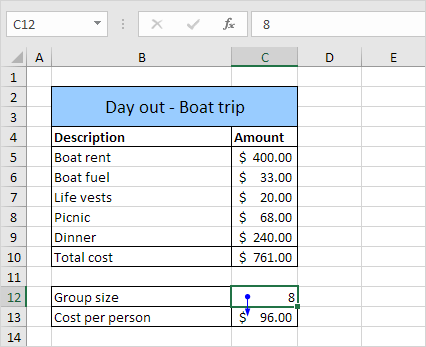As expected, the Cost per person depends on the Group size.

### Show Formulas

By default, Excel shows the results of formulas. To show the formulas instead of their results, execute the following steps.

1. On the Formulas tab, in the Formula Auditing group, click Show Formulas.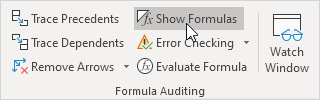Result: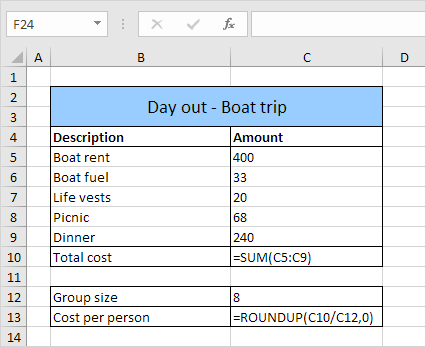Note: instead of clicking Show Formulas, press CTRL + ` (you can find this key above the tab key).

### Error Checking

To check for common errors that occur in formulas, execute the following steps.

1. Enter the value 0 into cell C12.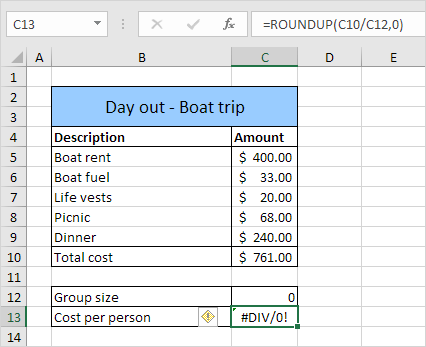2. On the Formulas tab, in the Formula Auditing group, click Error Checking.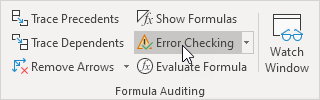Result. Excel finds an error in cell C13. The formula tries to divide a number by 0.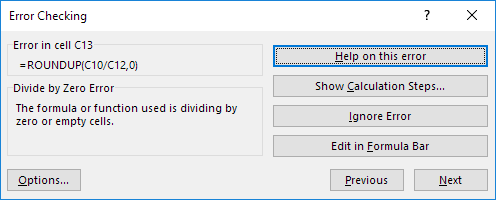### Evaluate Formula

To debug a formula by evaluating each part of the formula individually, execute the following steps.

1. Select cell C13.

2. On the Formulas tab, in the Formula Auditing group, click Evaluate Formula.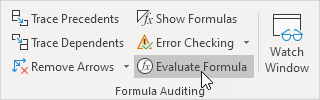3. Click Evaluate four times.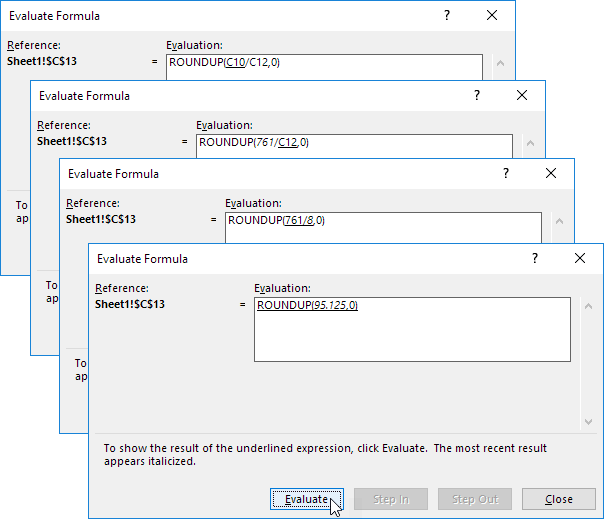Excel shows the formula result.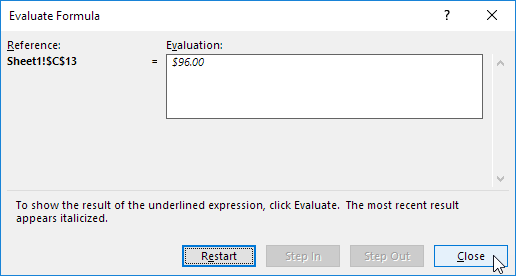Go to Next Chapter: Array Formulas